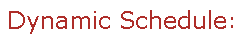Visit Minerva Class Schedule for course dates & times.

# MATH 356 Honours Probability (3 credits)

Offered by: Mathematics and Statistics (Faculty of Science)

### Overview

Mathematics & Statistics (Sci) : Sample space, probability axioms, combinatorial probability. Conditional probability, Bayes' Theorem. Distribution theory with special reference to the Binomial, Poisson, and Normal distributions. Expectations, moments, moment generating functions, uni-variate transformations. Random vectors, independence, correlation, multivariate transformations. Conditional distributions, conditional expectation.Modes of stochastic convergence, laws of large numbers, Central Limit Theorem.

Terms: Fall 2022

Instructors: Paquette, Elliot (Fall)

• Fall

• Prerequisite(s): MATH 243 or MATH 255, and MATH 222 or permission of the Department.

• Restriction: Not open to students who have taken or are taking MATH 323